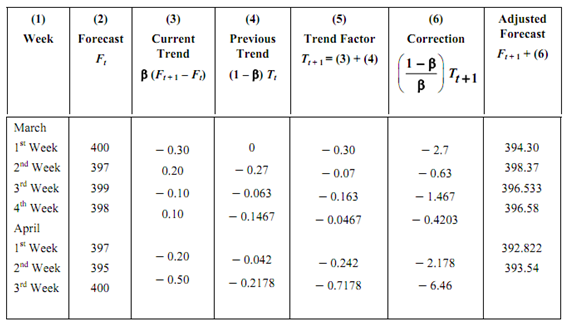## Example of simple exponential smoothing, Mechanical Engineering

Assignment Help:

Example of simple exponential smoothing:

A TV manufacturer utilizes simple exponential smoothing with α = 0.1 to forecast demand. For the first week of March, the forecast was 400 units while actual demand turned out to be 370 units.

1. Forecast the demand for the second week of March.

2. If the actual demand for the second week turned out to be 417 units, forecast the demand for the 3rd week. Continue forecasting for the subsequent four weeks. You can take actual demands for the subsequent weeks as 409, 388, 377, 445, and 410 units.

3. Develop an adjusted exponential forecast for this company. You may assume the initial trend adjustment factor Tt as zero and β = 0.1.

Solution

(a) Ft + 1 = Ft + α (Dt - Ft)

= 400 + 0.1 (370 - 400) = 397 units.

(b)(c)        The trend adjustment is accomplished by total of a correction factor

[(( 1 -β )/ β ) Tt +1 )]   to the simple exponential forecast.

As the initial trend adjustment factor (given) Tt = 0, we have

March 1st Week

Tt + 1 = β (Ft + 1 - Ft) + (1 - β) Tt

= 0.1 (397 - 400) + (0.9) (0) = - 0.30

T(t +1) adj = Ft +1 +( 1 -β / β )T t +1

= 397 +( 0.9/0.1)  (- 0.30) = 394.30

The adjusted forecast for the second week of March = 394.30.

March 2nd Week

Tt + 1 = 0.1 (399 - 397) + (0.9) (- 0.30)

= 0.2 - 0.27 = - 0.07

F(t + 1) adj = 399 + 9 (- 0.07) = 398.37

The remaining values may be put in table form, as below.The trend-adjusted forecast for the third week of April is Ft, adj   = 393.54 units whereas simple exponential forecast for the same week is 400 units.

The main reason for broad use of simple and adjusted exponential smoothing is that the forecast may be computerized quickly, requires very little memory space, and data can be updated easily. These methods are specifically useful to firms which have thousands of items in inventory. Since each forecast value contains all past forecast and demand data in the properly exponentially weighted manner, there is no requirement to maintain records of historical data (as in case of arithmetic moving averages). It is an effective and efficient method of forecasting with a built-in means of tracking the average while discounting the erratic random fluctuations.

#### Determine the isentropic flow through the nozzle, Determine the isentropic ...

Determine the isentropic flow through the nozzle: In an isentropic flow through the nozzle, air flows at rate of 600Kg/hr. At inlet to the nozzle, the pressure is 2Mpa and te

#### Lubrication, what are the necessity of lubrication ?

what are the necessity of lubrication ?

Home work

#### What do you mean by synchromesh device, What do you mean by synchromesh dev...

What do you mean by synchromesh device ? explain the basic working of a synchromesh gear box.

#### Calculate the survival function and expected value, Question Suppose T ...

Question Suppose T 1 and T 2 are independent and identically distributed Exponential variables with common hazard λ. What is the density of T = T 1 + T 2 ? What is the E(T-1

#### Evaluate the specific enthalpy - thermodynamics, Evaluate the specific enth...

Evaluate the specific enthalpy - thermodynamics: Steam is being generated in a boiler at a pressure of 15.25 bar. Calculate the specific enthalpy when ( i )  Steam i

#### proell governor, how to work proell govwrnor

how to work proell govwrnor?

#### Explain the information phase screw conveyor with auger, Information phase ...

Information phase of screw conveyor with auger: Steps: To secure all and complete information and gather facts and from best sources.Our project is selected based on the cu

#### Determine the bending stress, The cantilevered beam made from the Z section...

The cantilevered beam made from the Z section is subjected to the two loadings as shown. (a)  Determine and show the orientation of the neutral axis on the cross section (angle

#### Determine strain energy stored in coiled helical spring, Determine Strain e...

Determine Strain energy stored in coiled helical spring: For a close coiled helical spring subjected to an axial load of 200 N with 10 coils of wire of diameter 18 mm and buil